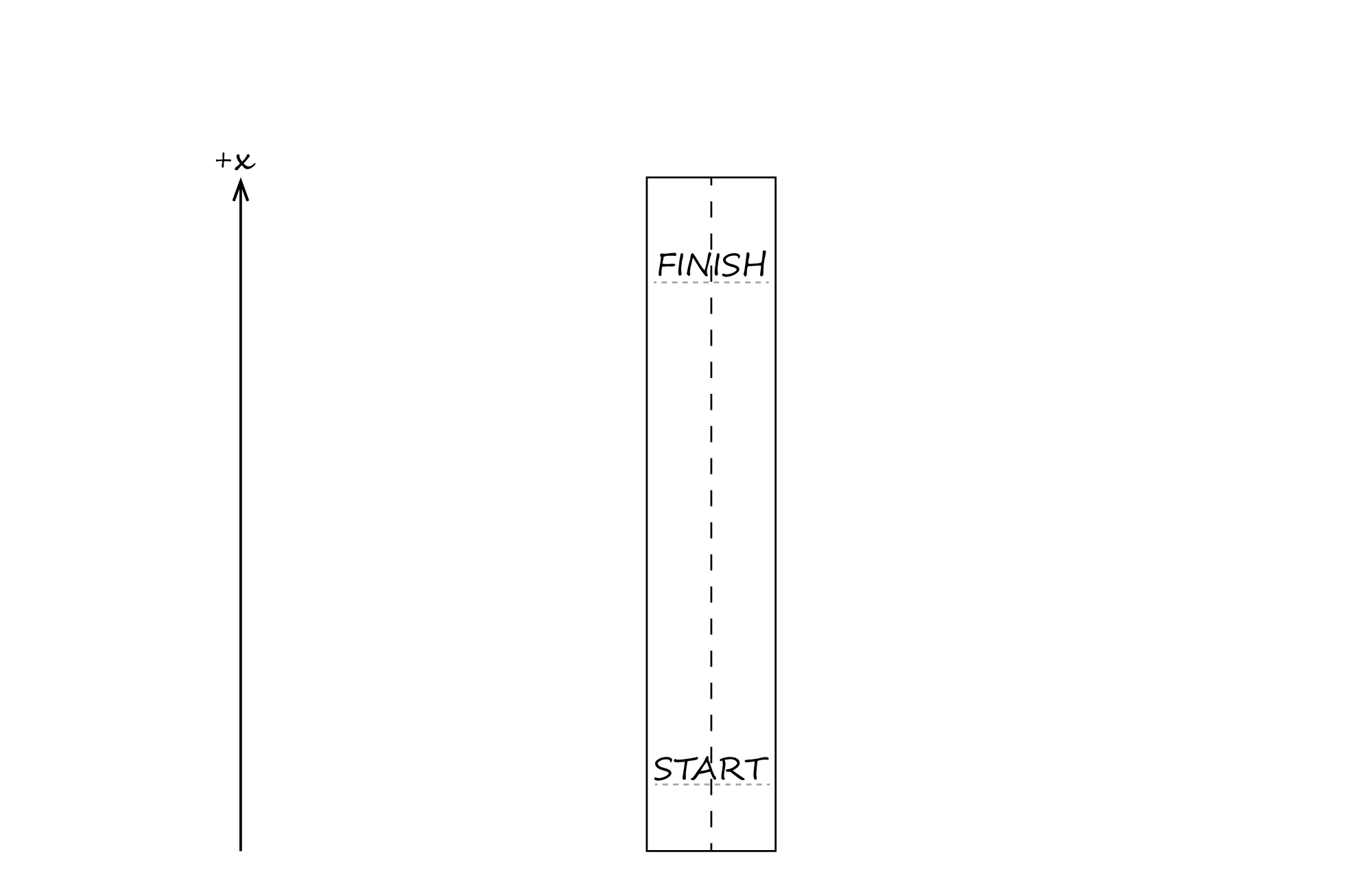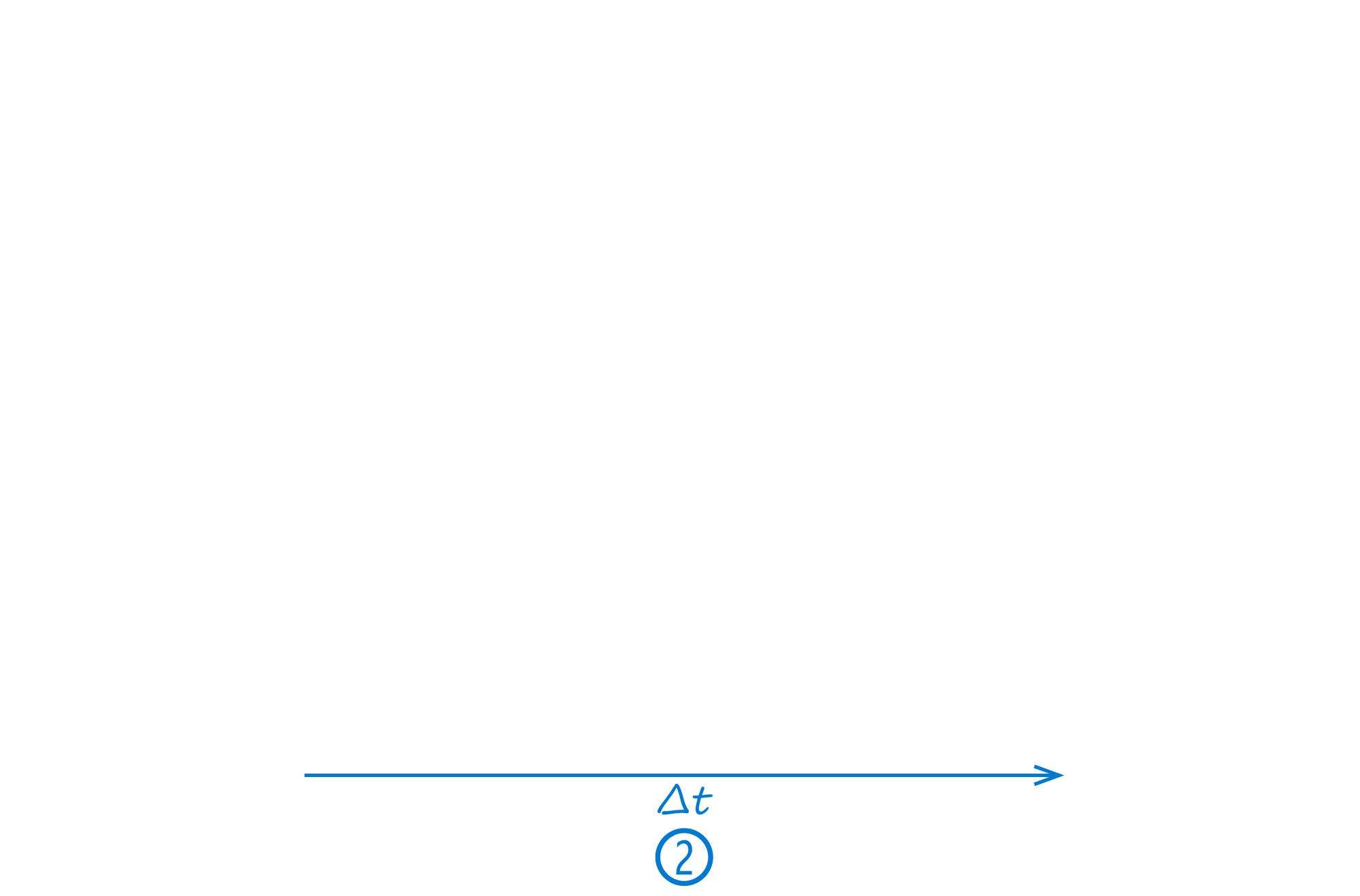DAVID LIAO.COM

## SiQuENC-REASoN table (references on homepage)

Si1. Let the +𝑥 direction be the direction of motion from the start line to the finish line of a straight racetrack. Si2. At the start of a race, Si3. Car A and Car B are stopped at the start line. Si4. Each car almost immediately gets up to speed and then continues to move at constant velocity Si5. through the end of the race, Si6. which happens when Car A crosses the finish line before Car B does.

Si7. Indicate whether Car A's average 𝑥‑velocity during the race is greater than, less than, or the same as Car B's average 𝑥‑velocity during the race.

Greater than Less than Same as

Example visibility settings
If not masked, make all parts or
Neatly and graphically represent situation(s) Graphically represent quantities and their relationships Identify relevant allowed starting point (in)equation(s) Use numbered steps to show REASoNing CommunicateQu1. We graphically represented 𝑥‑positions and 𝑥‑velocity vectors near our sketch. Qu2. We note a duration of elapsed time $\Delta t$ and draw 𝑥‑displacement vectors. Qu3. We can also draw an 𝑥‑position vs. time graph separate from the sketch of the situations.Qu4. The slope of a line connecting two points on an 𝑥‑position vs. time graph is the average 𝑥‑velocity of the motion beginning and ending at the times and 𝑥‑positions represented by those two points.

E1. Representations of (average) 𝑥‑velocity are showcased in the problem statement, our sketch of the situations, and our graph, so we write down an equation defining average 𝑥‑velocity.

 ④ $$\smash{\mathop{\uparrow}_{\mathrm{B}}^{\mathrm{A}}}\\[0pt]$$ $$\smash{v_{x,\mathrm{AVG}} \mathrel{\unicode{x2254}} \frac{\Delta x}{\Delta t}}$$ ① $$\smash{\mathop{\uparrow}_{\mathrm{B}}^{\mathrm{A}}}\\[2pt]$$ ③ $$\smash{\mathop{=}_{\mathrm{B}}^{\mathrm{A}}}\\[-6pt]$$ ②
Indicate and walk through relevant relationship(s) from allowed knowledge N1. N2. C1. According to the definition of average 𝑥‑velocity, average 𝑥‑velocity is proportional to 𝑥‑displacement and inversely proportional to elapsed time.
Indicate quantities that are equal and why they are equal N3. N4. N5. C2. During the race, Car A and Car B travel for the same amount of time because (a) the cars begin speeding up at the same time and (b) the race ends for both cars at the same time.
Indicate quantities that are altered or different and why they are altered or different N6. N7. N8. C3. Car A's 𝑥‑displacement is greater than Car B's 𝑥‑displacement because Car A crosses the finish line first.
So what? N9. N10. N11. C4. So, Car A's average 𝑥‑velocity during the race must be greater than Car B's average 𝑥‑velocity during the race. C5. (We check the option indicating "greater than").
Is there any quantity to analyze next? N12. No.